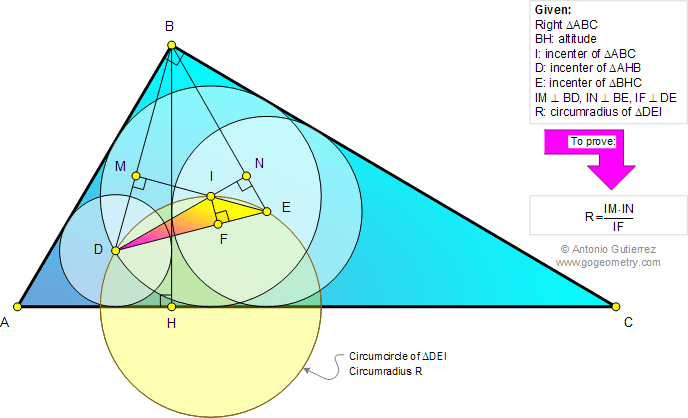# Online Geometry Problem 793: Right Triangle, Altitude, Three Incircles, Incenters, Circumcircle, Circumradius, Perpendicular, Distance, Metric Relations. Level: High School, SAT Prep, College, Mathematics Education

 The figure below shows a right triangle ABC with the incenter I and the altitude BH. D and E are the incenters of triangles AHB and BHC, respectively. IM, IN, and IF are perpendicular to BD, BE, and DE, respectively. If R is the circumradius of triangle DEI, prove that.Home | Search | Geometry | Problems | All Problems | Open Problems | Visual Index | 10 Problems | 791-800 | Triangles | Right Triangle | Circles | Incenter | Circumcirce / Circumradius | Altitude | Right triangle, Altitude and Incircle | Perpendicular | Email | Solution / comment | By Antonio Gutierrez